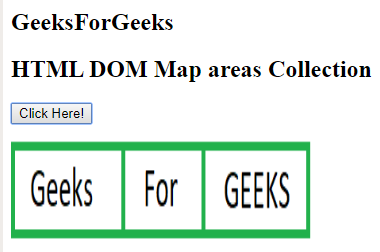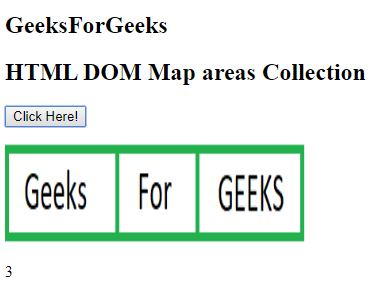Related Articles
HTML | DOM Map areas Collection
• Last Updated : 18 Nov, 2019

The Map areas Collection in HTML DOM is used to return the collection of all <area> elements in an image-map. The area elements are sorted as they appear in the source code.

Syntax:

`mapObject.areas`

Properties: It returns the number of area in the collection of elements.

Methods: The DOM Map areas Collection contains three methods which are listed below:

• [index]: It is used to return the <form> element of the specified index. The index value starts with 0. NULL value is returned if the index value is out of range.
• item(index): It is used to return the <form> element of specified index. The index value starts with 0. NULL value is returned if the index value is out of range. This method performs similarly to the above method.
• namedItem(id): It is used to return the <form> element from the collection which matches the specified id. NULL value is returned if the id does not exist.

Example:

 ` ``<``html``> `` ` `<``head``> ``    ``<``title``> ``        ``HTML DOM Map  areas Collection  ``    `` `` `` ` `<``body``> ``     ``<``h2``>``         ``GeeksForGeeks``     ````    ``<``h2``>HTML DOM Map  areas Collection ``     ` `    ``<``button` `onclick` `= ``"GFG()"``> ``        ``Click Here! ``    `` ``     ` `    ``<``p``> ``     ` `    ``<``map` `id` `= ``"Geeks"` `name` `= ``"Geeks"``> ``         ` `        ``<``area` `= ``"rect"` `coords` `= ``"0, 0, 110, 100"``        ``alt` `= ``"Geeks"` `href` `= ``"https://media.geeksforgeeks.org/wp-content/uploads/a1-21.png"``> `` ` `        ``<``area` `shape` `= ``"rect"` `coords` `= ``"110, 0, 190, 100"``        ``alt` `= ``"For"` `href` `= ``"https://media.geeksforgeeks.org/wp-content/uploads/a1-22.png"``> `` ` `        ``<``area` `shape` `= ``"rect"` `coords` `= ``"190, 0, 300, 100"``        ``alt` `= ``"GEEKS"` `href` `= ``"https://media.geeksforgeeks.org/wp-content/uploads/a1-24.png"``> ``    `` ``     ` `    ``<``img` `src` `= ``"https://media.geeksforgeeks.org/wp-content/uploads/a1-25.png"``    ``width` `= ``"300"` `height` `= ``"100"` `alt``=``"GFG"` `usemap` `= ``"#Geeks"``> ``     ` `    ``<``p` `id` `= ``"GEEK!"``> ``     ` `    ``<``script``> ``        ``function GFG() { ``            ``var x = document.getElementById("Geeks").areas.length; ``            ``document.getElementById("GEEK!").innerHTML = x; ``        ``} ``    `` ```

Output:

• Before Clicking the Button:• After Clicking the Button:Supported Browsers: The browsers supported by HTML DOM Map areas Collection are listed below:

• Google Chrome
• Internet Explorer
• Firefox
• Apple Safari
• OperaMy Personal Notes arrow_drop_up